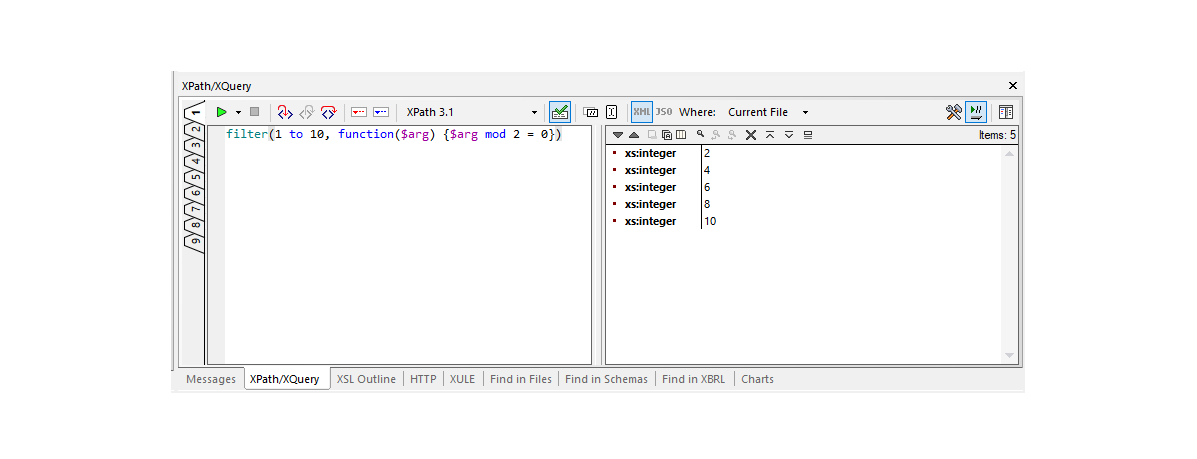## XPath/XQuery filter function

### Summary

Returns those items from the sequence \$seq for which the supplied function \$f returns true.

### Signature

`fn:filter`(
`\$seq` as `item()*`,
`\$f` as `function(item()) as xs:boolean`
) as `item()*`

### Properties

This function is deterministic, context-independent, focus-independent, and higher-order.

### Rules

The effect of the function is equivalent to the following implementation in XQuery:

```declare function fn:filter(
\$seq as item()*,
\$f as function(item()) as xs:boolean)
as item()* {
if (fn:empty(\$seq))
then ()
fn:filter(fn:tail(\$seq), \$f)
)
};```

or its equivalent in XSLT:

```<xsl:function name="fn:filter" as="item()*">
<xsl:param name="seq" as="item()*"/>
<xsl:param name="f" as="function(item()) as xs:boolean"/>
<xsl:if test="fn:exists(\$seq)">
<xsl:sequence select="fn:head(\$seq)[\$f(.) eq fn:true()], fn:filter(fn:tail(\$seq), \$f)"/>
</xsl:if>
</xsl:function>```

### Examples

The expression `fn:filter(1 to 10, function(\$a) {\$a mod 2 = 0})` returns `(2, 4, 6, 8, 10)`.

The expression `fn:filter((), fn:lang("en", ?))` returns `()`.### Error Conditions

As a consequence of the function signature and the function calling rules, a type error occurs if the supplied function \$f returns anything other than a single `xs:boolean` item; there is no conversion to an effective boolean value.

### Notes

The function call `fn:filter(\$SEQ, \$F)` has a very similar effect to the expression `\$SEQ[\$F(.)]`. There are some differences, however. In the case of `fn:filter`, the function `\$F` is required to return a boolean; there is no special treatment for numeric predicate values, and no conversion to an effective boolean value. Also, with a filter expression `\$SEQ[\$F(.)]`, the focus within the predicate is different from that outside; this means that the use of a context-sensitive function such as `fn:lang#1` will give different results in the two cases.